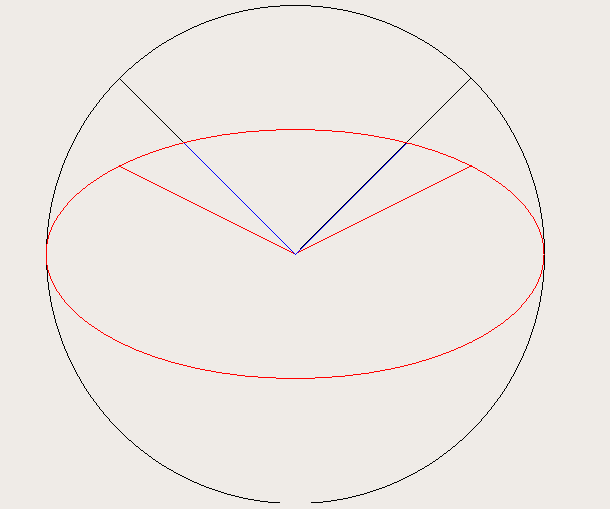# QPainter drawPie error in startAngle

• I am using `QPainter` to plot ellipses and segments of it (pies). I essentially use `QPainter::drawEllipse` and `QPainter::drawPie`. I've created this small example (note that the whole widget is not provided but only the paint event implementation):

``````void CustomWidget::paintEvent(QPaintEvent *event)
{
Q_UNUSED(event);
QPainter painter(this);

// Move painter to center
painter.translate(width() / 2, height() / 2);

// Get minimum size
double sz = std::min(width() / 2, height() / 2);

// Display circle
painter.drawEllipse(QPointF(0.0, 0.0), sz, sz);

// Display 45º
painter.drawPie(QRectF(-sz, -sz, 2 * sz, 2 * sz), 45 * 16, 0);

// Display 135º
painter.drawPie(QRectF(-sz, -sz, 2 * sz, 2 * sz), 135 * 16, 0);

// Change color
painter.setPen(QPen(Qt::red));

// Display ellipse
painter.drawEllipse(QPointF(0.0, 0.0), sz, sz / 2);

// Display 45º
painter.drawPie(QRectF(-sz, -sz / 2, 2 * sz, sz), 45 * 16, 0);

// Display 135º
painter.drawPie(QRectF(-sz, -sz / 2, 2 * sz, sz), 135 * 16, 0);

// Change color
painter.setPen(QPen(Qt::blue));

// Display real 45º on ellipse
double ang_real_45 = atan2(sin(45 * M_PI / 180) * sz, cos(45 * M_PI / 180) * sz / 2) * 180.0 / M_PI;
painter.drawPie(QRectF(-sz, -sz / 2, 2 * sz, sz), ang_real_45 * 16, 0);

// Display real 135 on ellipse
double ang_real_135 = atan2(sin(135 * M_PI / 180) * sz, cos(135 * M_PI / 180) * sz / 2) * 180.0 / M_PI;
painter.drawPie(QRectF(-sz, -sz / 2, 2 * sz, sz), ang_real_135 * 16, 0);
}
``````

Which outputs the following (ignore rendering issues and antialiasing):It can be seen how the lines that I draw from the center to the edge of the red ellipse using `QPainter::drawPie` are not at the right angle (45 and 135 degrees), and they end in the same x coordinate as the ones drawn on the black circle using the same functions. However, if we apply the scaling that we used to transform the circle into an ellipse, and recompute the angle, then the lines appear in the right place (blue lines). According to the documentation of `drawPie` :

Draws a pie defined by the given rectangle, startAngle and spanAngle.

The pie is filled with the current brush().

The startAngle and spanAngle must be specified in 1/16th of a degree, i.e. a full circle equals 5760 (16 * 360). Positive values for the angles mean counter-clockwise while negative values mean the clockwise direction. Zero degrees is at the 3 o'clock position.

However, the reality is that the parameters `startAngle` and `spanAngle` do not respect the angular proportions and the same scaling that was applied to the circle to convert it to an ellipse is applied, modifying the start and spawn angles. My questions are:

1- Am I doing something wrong?
2- Is this a bug? Should it be reported?
3- Why is this misleading behaviour not documented in the function documentation?
4- Can I submit this somewhere so this is at least incorporated in the documentation?

Thank you